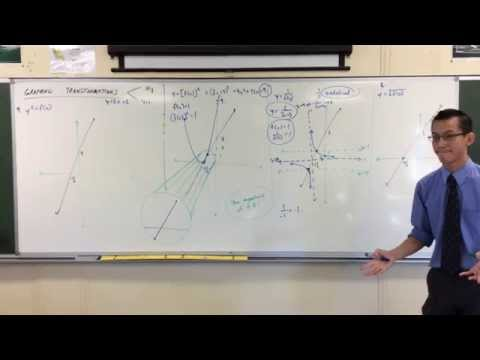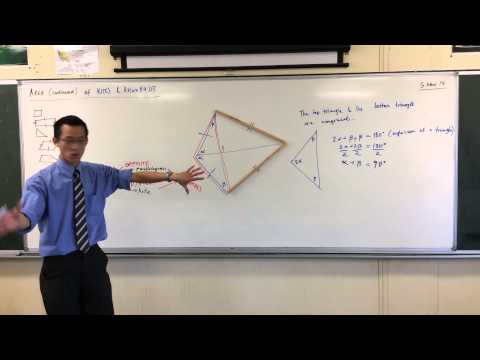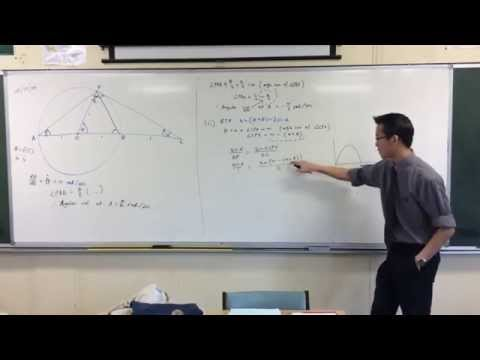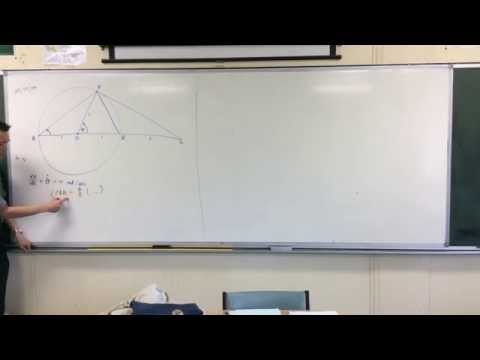### Showing videos fromEddie Woo with a total of 3,931 videos

03:35Basic Graphing Transformations (4 of 4: Square Root of a Function)
4y3m ago
09:15Basic Graphing Transformations (3 of 4: Squares & Reciprocals)
4y3m ago
11:28The Bridges of Königsberg (3 of 3: Understanding basic network theory)
4y4m ago
03:19The Bridges of Königsberg (2 of 3: Digging deeper into the problem)
4y4m ago
06:33Introduction to Paradoxes (2 of 3: Trigonometric Fallacy)
4y4m ago
08:10Traverse (Offset) Surveys (1 of 2: How to make the survey)
4y4m ago
11:46Areas of Kites & Rhombuses (2 of 2: Deriving the Formula)
4y4m ago
08:46Areas of Kites & Rhombuses (1 of 2: Understanding the Shape)
4y4m ago
11:52Areas of Plane Shapes (2 of 3: Triangles & Parallelograms)
4y4m ago
11:55The RSA Encryption Algorithm (2 of 2: Generating the Keys)
4y4m ago
08:40The RSA Encryption Algorithm (1 of 2: Computing an Example)
4y4m ago
07:04Mathematical Cryptosystems (2 of 2: Asymmetric Cryptography)
4y4m ago
07:33Mathematical Cryptosystems (1 of 2: Symmetric Cryptography)
4y4m ago
09:20Measures of Central Tendency (2 of 2: Quantitative & Categorical Data)
4y4m ago
15:26Measures of Central Tendency (1 of 2: Comparing Mean & Median)
4y4m ago
08:11The Unbreakable Cipher: One-Time Pads (2 of 2: Their Weaknesses)
4y4m ago
12:09The Unbreakable Cipher: One-Time Pads (1 of 2: How do they improve on Caesar?)
4y4m ago
07:05Calculating Heights w/ a Spreadsheet (3 of 3: How do you work out inches?)
4y4m ago
07:22Calculating Heights w/ a Spreadsheet (2 of 3: Converting metres to feet)
4y4m ago
08:59Calculating Heights w/ a Spreadsheet (1 of 3: Setting up the data)
4y4m ago
02:46Upgrading the Tax Spreadsheet (3 of 3: Extending to Multiple Tax Brackets)
4y4m ago
05:49Upgrading the Tax Spreadsheet (2 of 3: Distinguishing Tax Brackets)
4y4m ago
08:58Upgrading the Tax Spreadsheet (1 of 3: Introduction to IF Statements)
4y4m ago
05:49Calculating Income Tax w/ a Spreadsheet (2 of 2: Tax Payable)
4y4m ago
06:44Calculating Income Tax w/ a Spreadsheet (1 of 2: Annual Income)
4y4m ago
05:09Introduction to Cryptography (2 of 2: How to crack the Caesar Cipher)
4y4m ago
11:59Interesting Circle Geometry / Motion Question (2 of 2: External Angular Velocity)
4y4m ago
06:27Interesting Circle Geometry / Motion Question (1 of 2: Internal Angular Velocities)
4y4m ago
10:12Interesting Complex Polynomial Question (2 of 2: Trigonometric Result)
4y4m ago
14:33Interesting Complex Polynomial Question (1 of 2: Factorisation)
4y4m ago
07:04Calculating Compound Interest with a Spreadsheet (2 of 2: How to fix the formulas)
4y4m ago
13:22Calculating Compound Interest with a Spreadsheet (1 of 2: What's wrong?)
4y4m ago
10:35Matrix Determinants (2 of 3: The Determinant's Geometric Meaning)
4y5m ago